The function buildAxis is built to provide more control of the number of labels on the axis. This function is still under development.

## Usage

buildAxis(side, limits, n, nMin = 2, nMax = 10, extend = 2, eps = 10^-12, ...)

## Arguments

side

The side of the plot where to add the axis.

limits

Either lower and upper limits on the axis or a data set.

n

The preferred number of axis labels.

nMin

The minimum number of axis labels.

nMax

The maximum number of axis labels.

extend

How far the axis may extend beyond range(limits).

eps

The smallest increment allowed.

...

Arguments passed to axis

## Value

A vector of the axis plotted.

## Details

The primary reason behind building this function was to allow a plot to be created with similar features but with different data sets. For instance, if a set of code was written for one data set and the function axis had been utilized with pre-specified values, the axis may not match the plot of a new set of data. The function buildAxis addresses this problem by allowing the number of axis labels to be specified and controlled.

The axis is built by assigning penalties to a variety of potential axis setups, ranking them based on these penalties and then selecting the axis with the best score.

histPlot, dotPlot, boxPlot, densityPlot

David Diez

## Examples


# ===> 0 <===#
limits <- rnorm(100, 605490, 10)
hist(limits, axes = FALSE)
buildAxis(1, limits, 2, nMax = 4)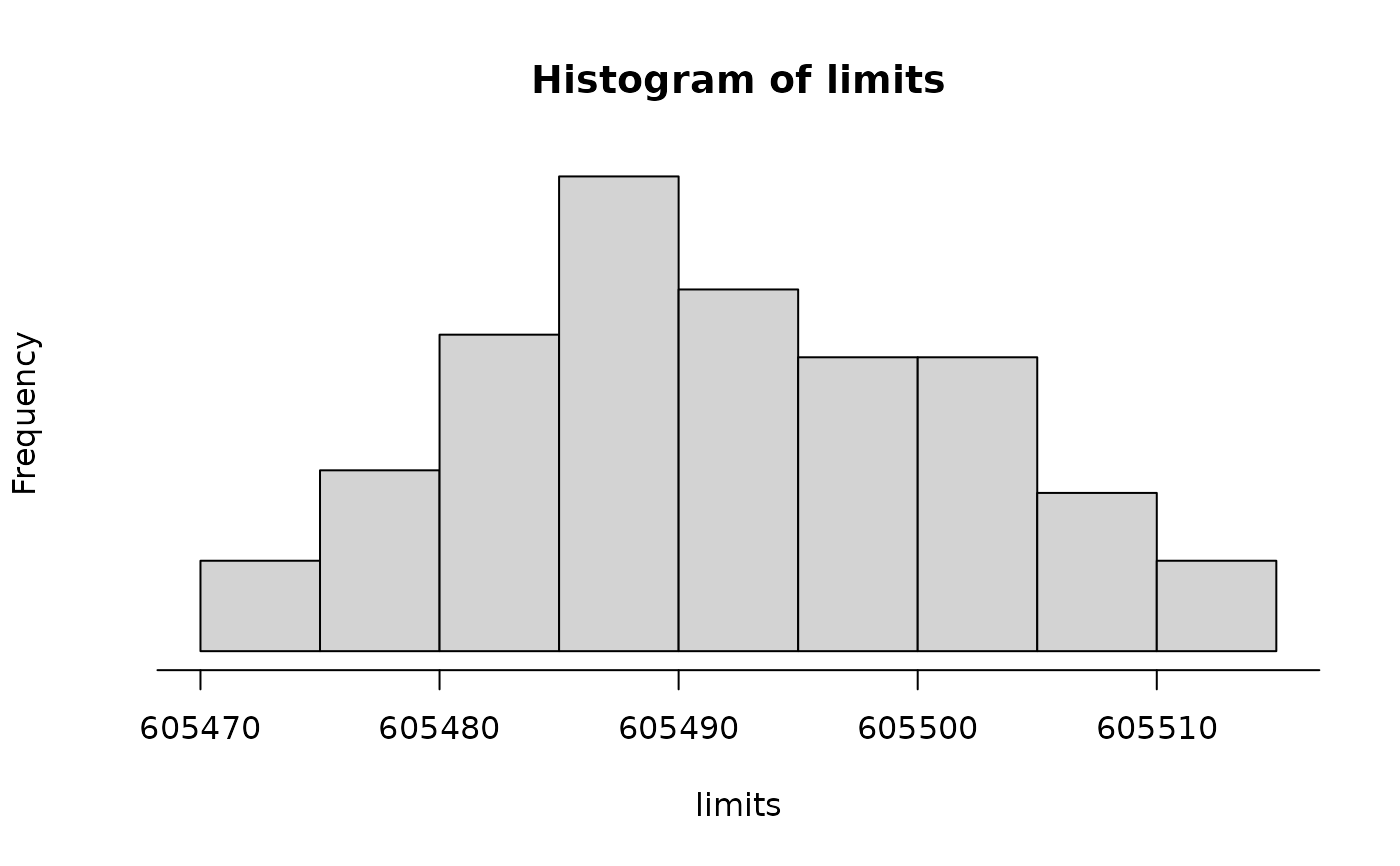# ===> 1 <===#
x <- seq(0, 500, 10)
y <- 8 * x + rnorm(length(x), mean = 6000, sd = 200)
plot(x, y, axes = FALSE)
buildAxis(1, limits = x, n = 5)
buildAxis(2, limits = y, n = 3)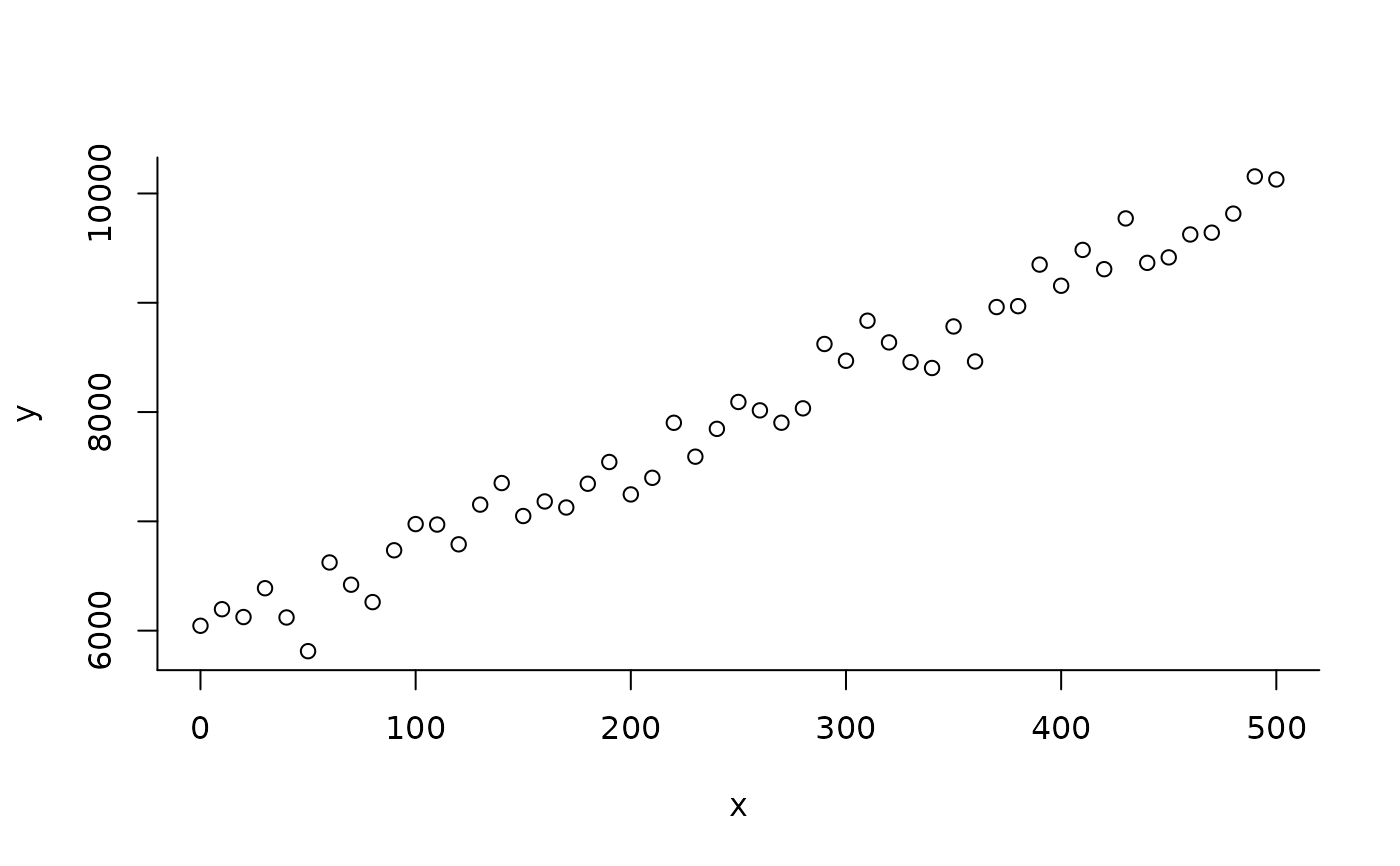# ===> 2 <===#
x <- 9528412 + seq(0, 200, 10)
y <- 8 * x + rnorm(length(x), mean = 6000, sd = 200)
plot(x, y, axes = FALSE)
temp <- buildAxis(1, limits = x, n = 4)
buildAxis(2, y, 3)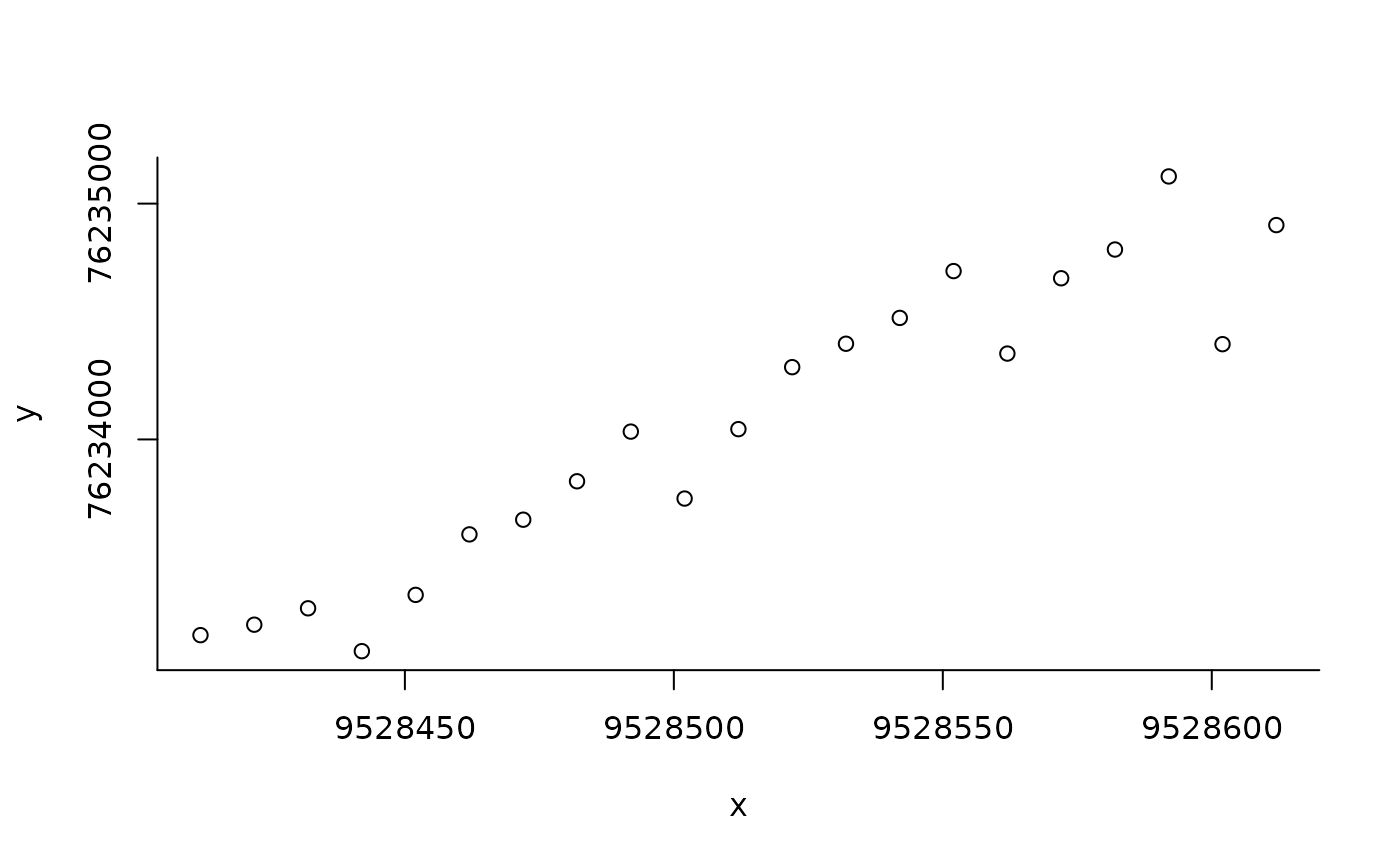# ===> 3 <===#
x <- seq(367, 1251, 10)
y <- 7.5 * x + rnorm(length(x), mean = 6000, sd = 800)
plot(x, y, axes = FALSE)
buildAxis(1, limits = x, n = 4, nMin = 3, nMax = 3)
buildAxis(2, limits = y, n = 4, nMin = 3, nMax = 5)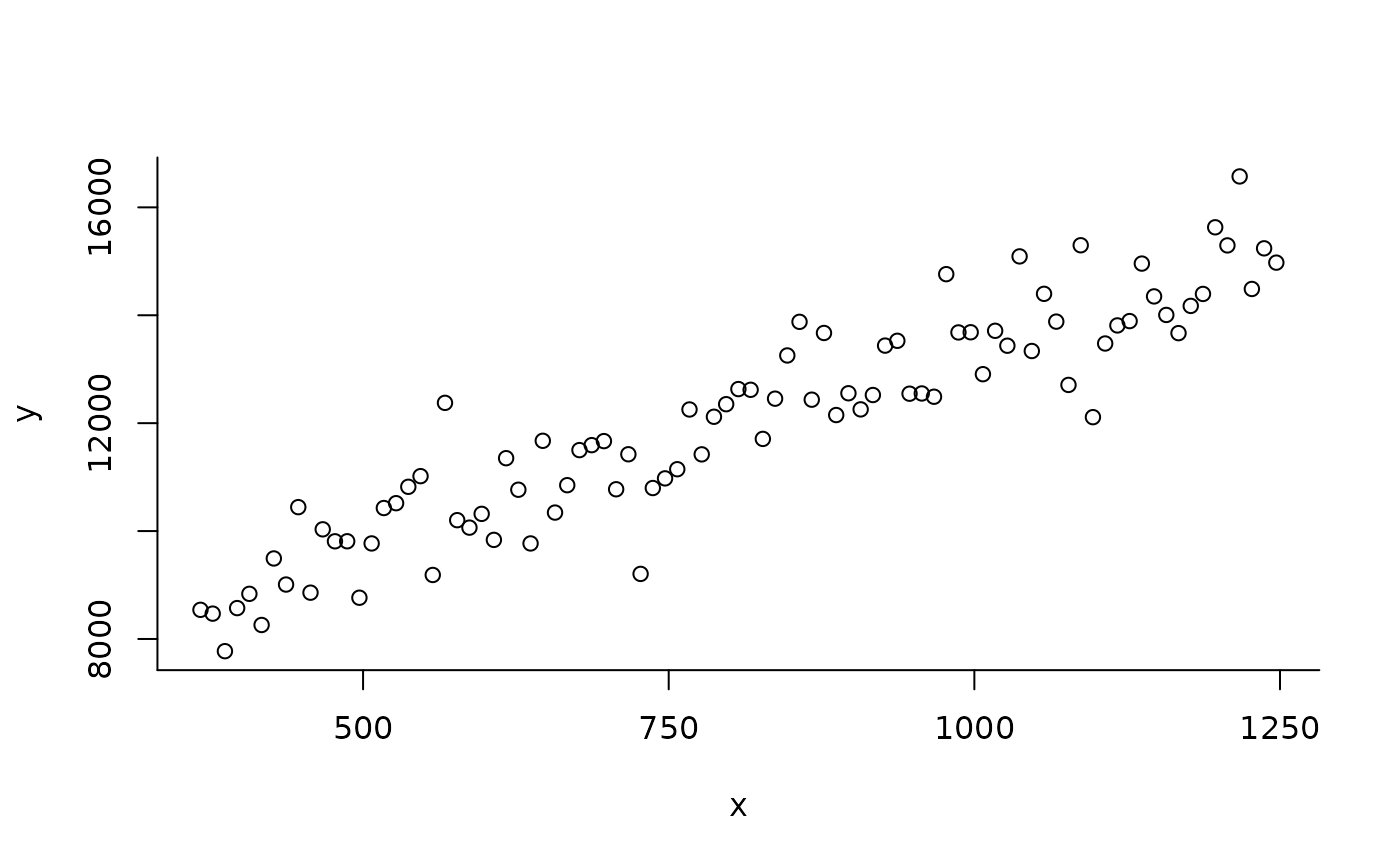# ===> 4 <===#
x <- seq(367, 367.1, 0.001)
y <- 7.5 * x + rnorm(length(x), mean = 6000, sd = 0.01)
plot(x, y, axes = FALSE)
buildAxis(1, limits = x, n = 4, nMin = 5, nMax = 6)
buildAxis(2, limits = y, n = 2, nMin = 3, nMax = 4)# ===> 5 <===#
x <- seq(-0.05, -0.003, 0.0001)
y <- 50 + 20 * x + rnorm(length(x), sd = 0.1)
plot(x, y, axes = FALSE)
buildAxis(1, limits = x, n = 4, nMin = 5, nMax = 6)
buildAxis(2, limits = y, n = 4, nMax = 5)
abline(lm(y ~ x))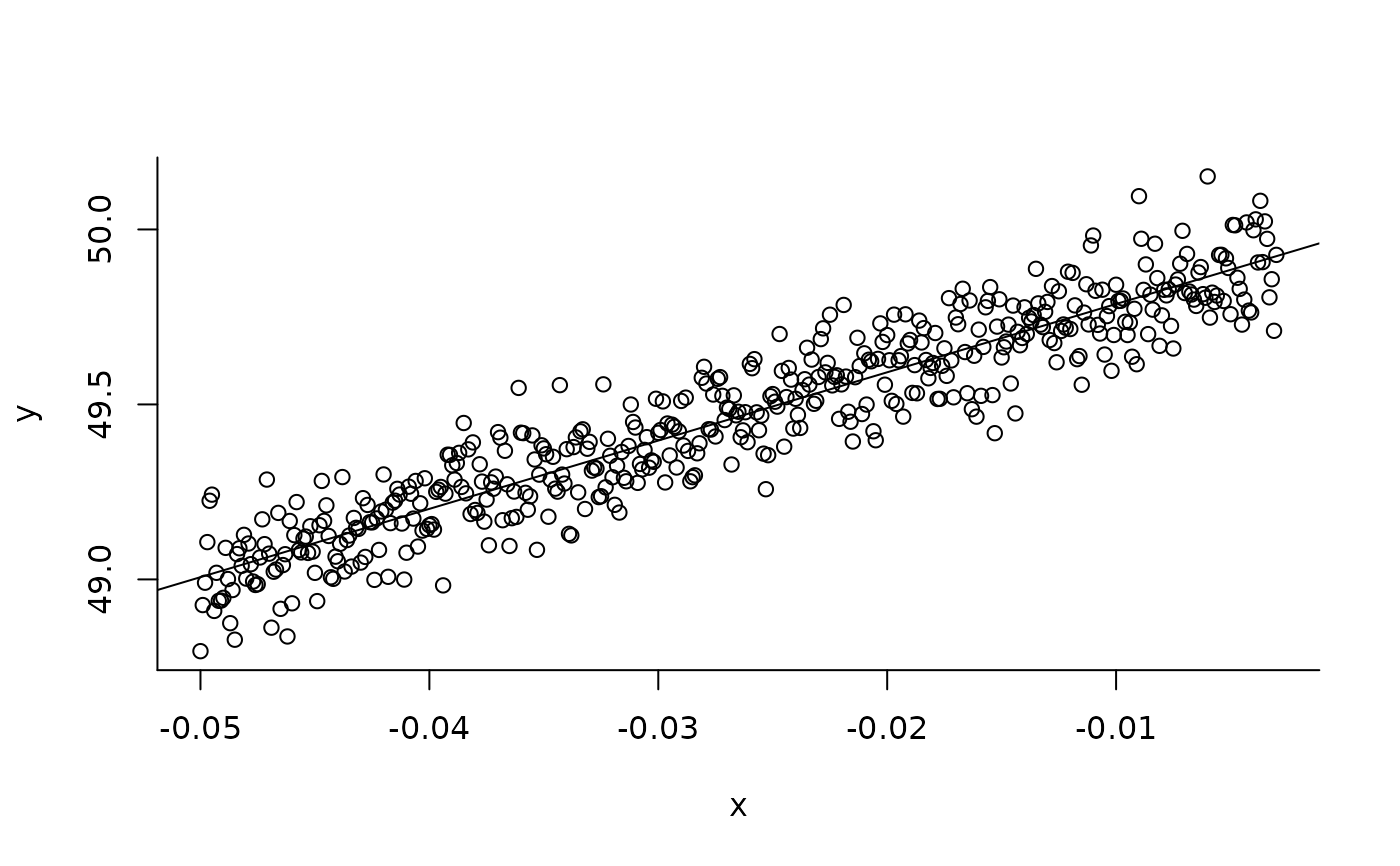# ===> 6 <===#
x <- seq(-0.0097, -0.008, 0.0001)
y <- 50 + 20 * x + rnorm(length(x), sd = 0.1)
plot(x, y, axes = FALSE)
buildAxis(1, limits = x, n = 4, nMin = 2, nMax = 5)
buildAxis(2, limits = y, n = 4, nMax = 5)
abline(lm(y ~ x))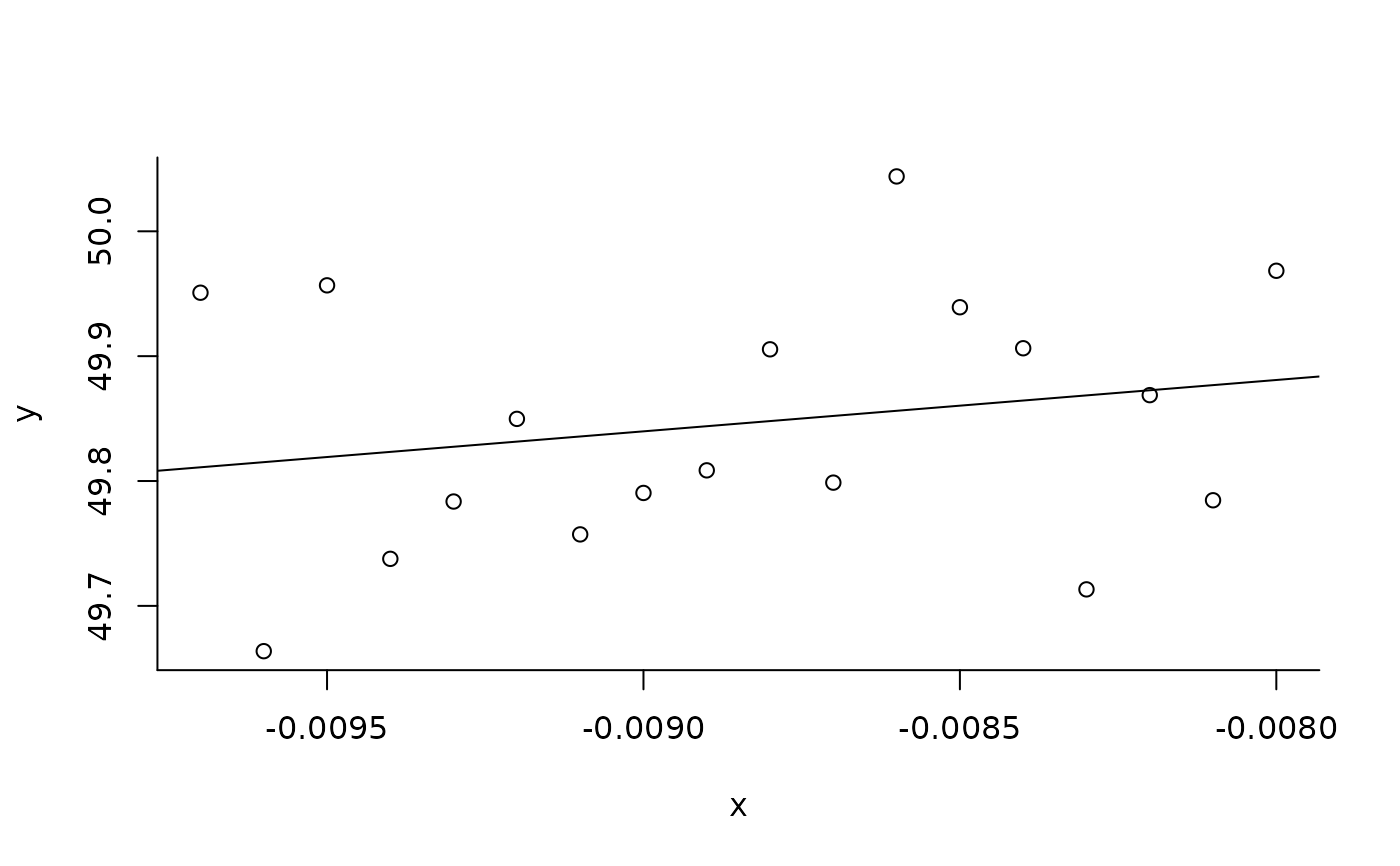# ===> 7 <===#
x <- seq(0.03, -0.003099, -0.00001)
y <- 50 + 20 * x + rnorm(length(x), sd = 0.1)
plot(x, y, axes = FALSE)
buildAxis(1, limits = x, n = 4, nMin = 2, nMax = 5)
buildAxis(2, limits = y, n = 4, nMax = 6)
abline(lm(y ~ x))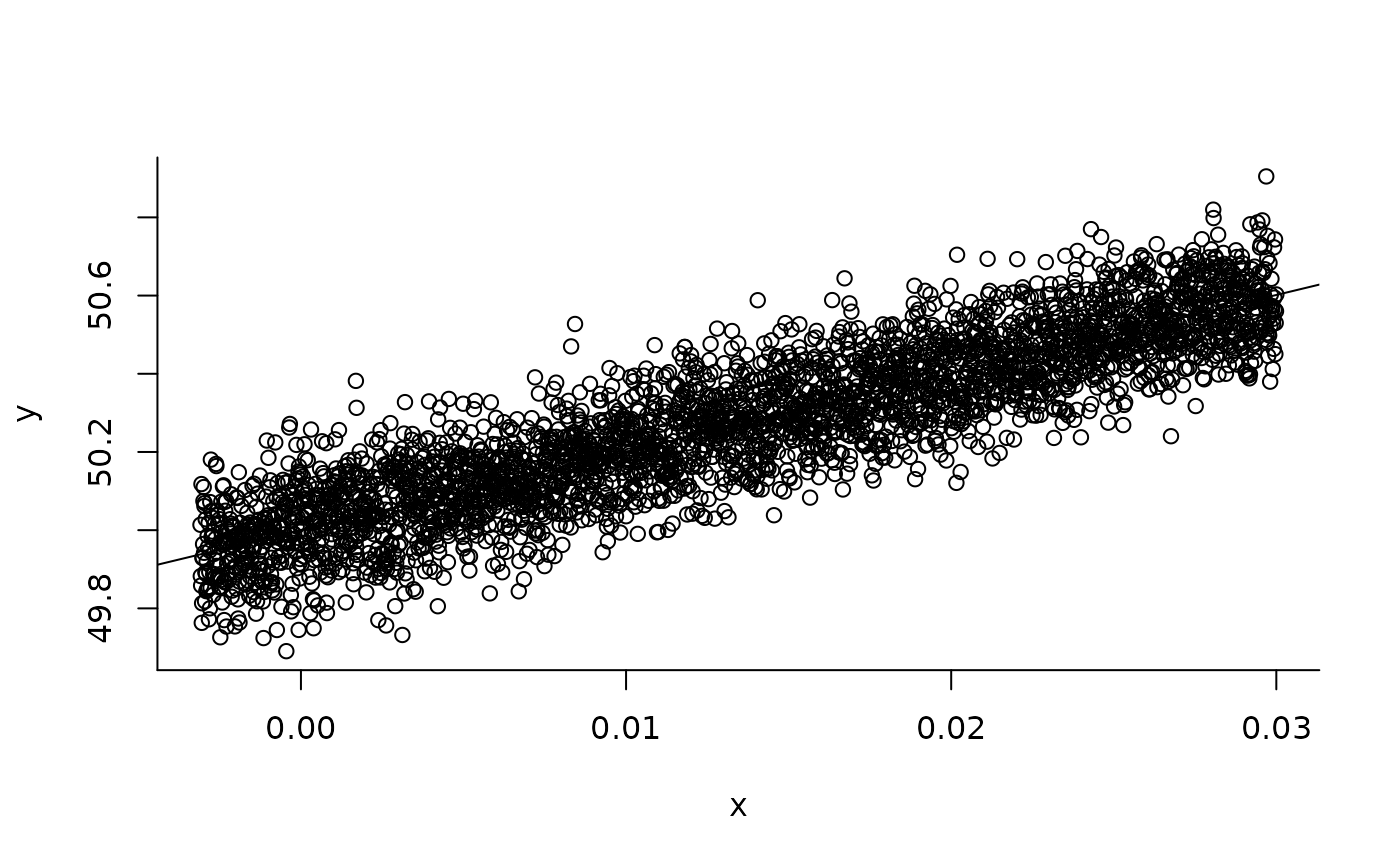# ===> 8 - repeat <===#
m <- runif(1) / runif(1) +
rgamma(1, runif(1) / runif(1), runif(1) / runif(1))
s <- rgamma(1, runif(1) / runif(1), runif(1) / runif(1))
x <- rnorm(50, m, s)
hist(x, axes = FALSE)
buildAxis(1, limits = x, n = 5, nMin = 4, nMax = 6, eps = 10^-12)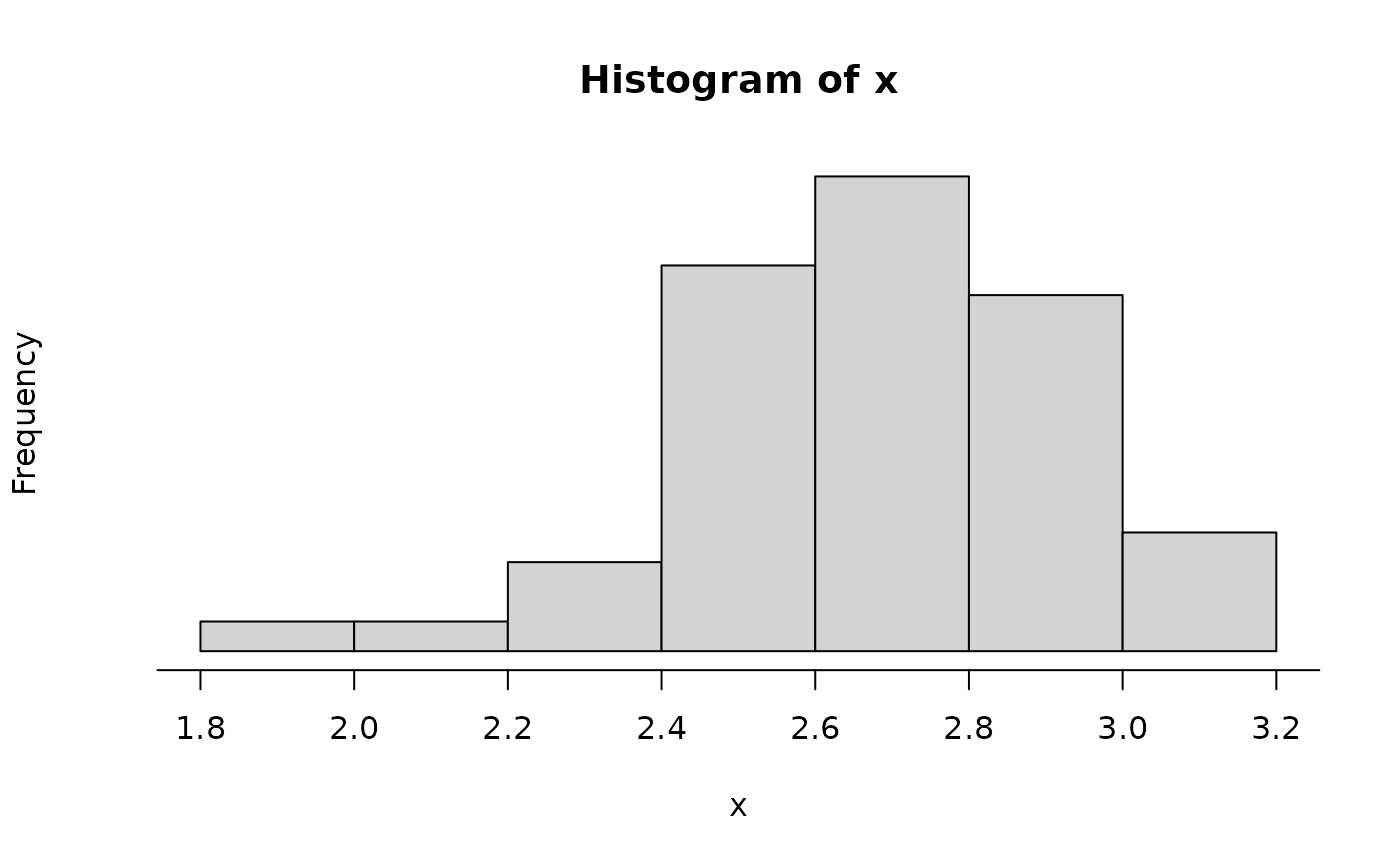if (diff(range(x)) < 10^-12) {
cat("too small\n")
}